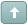Error

 Previous Topic Next Topic
 Ted #1 Posted : Saturday, September 18, 2004 1:29:49 PM(UTC)Rank: NewbieGroups: Registered, Registered Users, SubscribersJoined: 9/18/2004(UTC)Posts: 1 does anyone have two-day swing chart formula? much appreciated!Wanna join the discussion?! Login to your Discussions forum accountor Register a new forum account.Patrick #2 Posted : Saturday, September 18, 2004 4:28:33 PM(UTC)Rank: Advanced MemberGroups: Registered, Registered Users, SubscribersJoined: 9/8/2004(UTC)Posts: 2,266Was thanked: 1 time(s) in 1 post(s) This is the formula for a Gann Swing : {Market swing is defined as: Up = 2 higher highs, Down = 2 lower highs.} Us:=BarsSince((H > Ref(H,-1)) AND (Ref(H,-1) > Ref(H,-2))); Ds:=BarsSince((L < Ref(L,-1)) AND (Ref(L,-1) < Ref(L,-2))); Sd1:=If(Us=0, {then}If(Ref(L,-1)<>LowestSince(1,Ds=0,L), {then}1, {else}0), {else}If(Ds=0, {then}If(Ref(H,-1)<> HighestSince(1,Us=0,H), {then}-1, {else}0), {else}0)); Sd2:=If(Sd1=1, {then} If(Ref(BarsSince(Sd1=1),-1) > Ref(BarsSince(Sd1=-1),-1), {then}1, {else}0), {else} If(Sd1=-1, {then}If(Ref(BarsSince(Sd1=1),-1) < Ref(BarsSince(Sd1=-1),-1), {then}-1, {else}0), {else}0)); TD1:=ValueWhen(1,Sd2<>0,Sd2); Td1; This is only an indicator that will go from 1 to -1. Ultimately I would create an expert using this indicator and the cross function, which will make a lot more sense than an indicator. If that is not what you wanted then let me know more info, I might be able to create it for you.Patrick #3 Posted : Monday, September 20, 2004 6:37:39 PM(UTC)Rank: Advanced MemberGroups: Registered, Registered Users, SubscribersJoined: 9/8/2004(UTC)Posts: 2,266Was thanked: 1 time(s) in 1 post(s) If you want to draw level lines at the swing points, try using this : Us:=BarsSince((H>Ref(H,-1)) AND (Ref(H,-1)>Ref(H,-2))); Ds:=BarsSince((LLowestSince(1,Ds=0,L),1,0), If(Ds=0,If(Ref(H,-1)<>HighestSince(1,Us=0,H),-1,0),0)); Sd2:=If(Sd1=1,If(Ref(BarsSince(Sd1=1),-1)> Ref(BarsSince(Sd1=-1),-1),1,0),If(Sd1=-1, If(Ref(BarsSince(Sd1=1),-1)0,Sd2); Up:=Cross(TD1,.5); Dn:=Cross(-.5,TD1); If(BarsSince(up)>BarsSince(Dn),ValueWhen(1,DN,Ref(H,-1)),ValueWhen(1,UP,Ref(L,-1))) If you want to mark the bar when the swing occur ... You would have to create a new expert advisor and use the highlights or symbols. In the symbols (or highlight tab) Click on New and call it Up swing ( or whatever ). Select the symbol, the color and its position. In the formula Type : Us:=BarsSince((H>Ref(H,-1)) AND (Ref(H,-1)>Ref(H,-2))); Ds:=BarsSince((LLowestSince(1,Ds=0,L),1,0), If(Ds=0,If(Ref(H,-1)<>HighestSince(1,Us=0,H),-1,0),0)); Sd2:=If(Sd1=1,If(Ref(BarsSince(Sd1=1),-1)>Ref(BarsSince(Sd1=-1),-1),1,0), If(Sd1=-1,If(Ref(BarsSince(Sd1=1),-1)0,Sd2); Cross(TD1,.5) Then Click on new again, this time call it Down swing, Select the symbol, the color and its position. Us:=BarsSince((H>Ref(H,-1)) AND (Ref(H,-1)>Ref(H,-2))); Ds:=BarsSince((LLowestSince(1,Ds=0,L),1,0),If(Ds=0,If(Ref(H,-1)<> HighestSince(1,Us=0,H),-1,0),0)); Sd2:=If(Sd1=1,If(Ref(BarsSince(Sd1=1),-1)>Ref(BarsSince(Sd1=-1),-1),1,0),If(Sd1=-1, If(Ref(BarsSince(Sd1=1),-1)0,Sd2); Cross(-.5,TD1) Then attach the expert on your chart. The way I did mine, it plotted a red arrow above the price bar on a down swing day and a green arrow below the price bar on a upswing day. But you could use anything symbol you want or highlights instead.jack #4 Posted : Wednesday, February 16, 2005 1:18:23 AM(UTC)Rank: NewbieGroups: Registered, Registered Users, SubscribersJoined: 2/11/2005(UTC)Posts: 1Location: near Albany NY USA :) Hi Patrick, I downloaded the code for the upswing and the downswing that you gave. The upswing worked, but the downswing posted an error in code - "A "(" must immediately follow a function name". As I am a newcomer I admit I don't know where to look for the error. If you could help me understand I would appreciate it. Enjoy looking at the forum and codes. Thanks for the help you give . johnPatrick #5 Posted : Wednesday, February 16, 2005 3:15:26 PM(UTC)Rank: Advanced MemberGroups: Registered, Registered Users, SubscribersJoined: 9/8/2004(UTC)Posts: 2,266Was thanked: 1 time(s) in 1 post(s) In the last Line I wrote cross(.5,Swing) There is no swing variable anywhere in the formula and it is a function. I had to replace it by Cross(.5,TD1) It is fixed in the post above, let me know if you run into other problems. Glad to hear you enjoy the Forum, I appreciate it :DUsers browsing this topic
Forum Jump
You cannot post new topics in this forum.
You cannot reply to topics in this forum.
You cannot delete your posts in this forum.
You cannot edit your posts in this forum.
You cannot create polls in this forum.
You cannot vote in polls in this forum.